# NCERT solutions for class 9 maths chapter 5 Ex 5.1 and 5.2

In this page we have NCERT solutions for class 9 maths chapter 5 Euclids Geometry for EXERCISE 5.1 & 5.2 on pages 85,86 and 88. Hope you like them and do not forget to like , social share and comment at the end of the page.

## Chapter 5 Ex 5.1

Question 1:
Which of the following statements are true and which are false? Give reasons for your answers.
(i) Only one line can pass through a single point.
(ii) There are an infinite number of lines which pass through two distinct points.
(iii) A terminated line can be produced indefinitely on both the sides.
(iv) If two circles are equal, then their radii are equal.
(v) In below figure, if AB = PQ and PQ = XY, then AB = XY.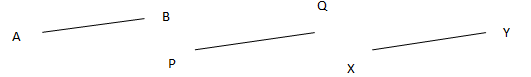Solution:
(i) False. Since through a single point, infinite number of lines can pass
(ii) False. Since through two distinct points, only one line can pass
(iii) True. A terminated line can be produced indefinitely on both the sides.
(iv)True. If two circles are equal, then their centre and circumference will coincide and hence, the radii will also be equal.
(v) True. It is given that AB and XY are two terminated lines and both are equal to a third line PQ. Euclid’s first axiom states that things which are equal to the same thing are equal to one another. Therefore, the lines AB and XY will be equal to each other.

Question 2:
Give a definition for each of the following terms. Are there other terms that need to be
defined first? What are they, and how might you define them?
1. parallel lines
2. perpendicular lines
3. line segment
5. square
Solution:
(i) Parallel Lines:
If the perpendicular distance between two lines is always constant, these are called parallel lines. In other words we may say that the lines which never intersect each other are called parallel lines.
To define parallel line, we must know about point, lines and distance between lines and point of intersection.
The definitions of line, point, plane explained by Euclid is not accepted by the Mathematician. So these terms are taken as undefined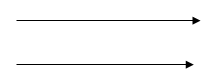(ii) Perpendicular lines:
If two lines intersect each other at 90° , these are called perpendicular lines. We need to define line and the angle before defining perpendicular lines.
The definitions of line, point, plane explained by Euclid is not accepted by the Mathematician. So these terms are taken as undefined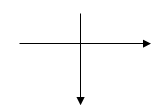(iii) Line segment:
A straight line drawn from any point to any other point is called as line segment. To define line segment, we must know about point and line segment.
The definitions of line, point, plane explained by Euclid is not accepted by the Mathematician. So these terms are taken as undefined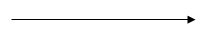It is the distance between the centre of a circle to any point lying on the circle. To define radius of circle, we must know about point and circle.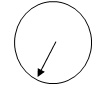The definitions of line, point, plane explained by Euclid is not accepted by the Mathematician. So these terms are taken as undefined

(v) Square:
A square is a quadrilateral having all sides of equal length and all angles of same measure i.e. 90o . To define square, we must know about quadrilateral, side, and angle.
The definitions of line, point, plane explained by Euclid is not accepted by the Mathematician. So these terms are taken as undefined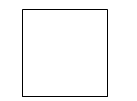Question 3
Consider two ‘postulates’ given below:
(i) Given any two distinct points A and B, there exists a third point C which is in between A and B.
(ii) There exist at least three points that are not on the same line.
Do these postulates contain any undefined terms? Are these postulates consistent?
Do they follow from Euclid’s postulates? Explain.
Solution
There are various undefined terms in the given postulates.
The given postulates are consistent because they refer to two different situations. Also, it is impossible to deduce any statement that contradicts any well-known axiom and postulate.
These postulates do not follow from Euclid’s postulates. They follow from the axiom, “Given two distinct points, there is a unique line that passes through them”.

Question 4:
If a point C lies between two points A and B such that AC = BC, then prove that
$AC =\frac {1}{2} AB$. Explain by drawing the figure.
Solution:
Given that from question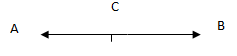AC=BC
We add equals on both sides
$AC+AC=BC+AC$ ... (a)
Here, (BC + AC) coincides with AB. We know that things which coincide with one another are equal to one another.
$BC + AC =AB$ ... (b)
We know that things which are equal to the same thing are equal to one another. So, from equation (a) and equation (b), we have
$AC + AC = AB$
$2AC = AB$
$AC =\frac {1}{2} AB$

Question 5:
In Question 4, point C is called a mid-point of line segment AB. Prove that every line
segment has one and only one mid-point.
Solution
Let us assume there are two mid-points $C_1$ and $C_2$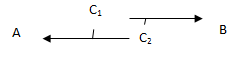$C_1$ is midpoint of AB
$AC_1 = C_1B$
$A C_1 + A C_1 = B C_1 + A C_1$ (equals are added on both sides)    ... (1)
Here, ($B C_1 + A C_1$) coincides with AB. We know that things which coincide with one another are equal to one another.
$B C_1 + A C_1 = AB$ ... (2)
We know that things which are equal to the same thing are equal to one another. So, from equation (1) and equation (2), we have
$A C_1 + A C_1 = AB$
$2A C_1= AB$... (3)
Similarly by taking $C_2$ as the mid-point of AB, we can prove that
$2A C_2 = AB$ ... (4)
From equation (3) and (4), we have
$2A C_1= 2A C_2$ (Things which are equal to the same thing are equal to one another.)
$A C_1 = A C_2$ (Things which are double of the same things are equal to one another.)
It is possible only when point $C_1$ and $C_2$ are representing a single point.
Hence our assumption is wrong and there can be only one mid-point of a given line segment.

Question 6:
If $AC = BD$, then prove that $AB = CD$.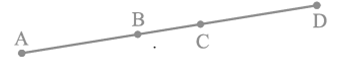Solution:
We can be observed from the figure that
$AC = AB + BC$
$BD = BC + CD$
It is given that AC = BD
So $AB + BC = BC + CD$
According to Euclid’s axiom, when equals are subtracted from equals, the remainders are also equal.
Subtracting BC from equation (1), we obtain
$AB + BC -BC = BC + CD - BC$
AB = CD

Question 7:
Why is Axiom 5, in the list of Euclid’s axioms, considered a ‘universal truth’? (Note that the question is not about the fifth postulate.)
Solution:
Axiom 5: The whole is greater than the part.
Let X is representing a whole quantity and only a, b, c are parts of it.
Now X = a + b + c
Clearly X will be greater than all its parts a, b and c
So, it is rightly said that the whole is greater than the part.
There can be many examples.  This is true for anything in any part of the world, this is a universal truth.

## Chapter 5 Ex 5.2

Question 1:
How would you rewrite Euclid's fifth postulate so that it would be easier to understand?
Solution:
Two distinct intersecting lines cannot be parallel to the same line

Question 2:
Does Euclid's fifth postulate imply the existence of parallel lines? Explain.
Solution:
Yes it is true
If a straight line falling on two straight lines makes the interior angles on the same side of it taken together less than two right angles, then the straight lines if produced indefinitely meet on that side on which the angles are less than the two right angles
If the sum interior angles on the same side of it taken together is equal to two right angles,then two lines will not meet on either side. Hence lines are parallel

## Summary

1. NCERT solutions for class 9 maths chapter 5 Euclid Geometry Exercise 5.1 and 5.2 has been prepared by Expert with utmost care. If you find any mistake.Please do provide feedback on mail. You can download the solutions as PDF in the below Link also
2. This chapter 5 has total 2 Exercise 5.1 and 5.2. This page has both the exercise in the chapter.

• Notes
• NCERT Solutions
• Assignments
• Revision sheet